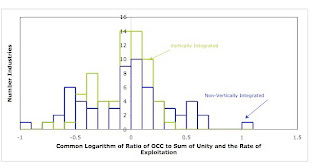## Monday, September 08, 2008

### OCC Varies Less Among Vertically Integrated Industries (Part 2)

I gave a hostage to fortune in the first part. That part notes the empirical claim that the Organic Composition of Capital (OCC) varies less among vertically integrated industries, as compared to non-vertically integrated industries. But I did not demonstrate this claim with actual data. This part retrieves this hostage by presenting empirical results.

The first part explained how to calculate the OCC for both non-vertically and vertically integrated industries, given Leontief Input Output tables. I performed these calculations with the Leontief Input Output table obtainable from the 2005 Use Table and other data available from the Bureau of Economic Analysis (BEA). Figure 1 shows these distributions of the OCC among the 65 industries aggregated by the BEA. Notice that the OCC does indeed seem to be more dispersed for non-vertically integrated industries.Figure 1: Distribution of Ratio of OCC to Sum ofUnity and Rate of Exploitation
In both cases, the distributions seem to be skewed and from a non-Gaussian distribution. Taking common logarithms yields the distributions shown in Figure 2. Table 1 presents summary statistics for these distributions. The absolute value of the coefficient of variation in the distribution of the OCC is indeed decreased by vertical integration. So these results replicate, for 2005 United States of America (USA) data, Shaikh’s and Petrovic’s earlier results for the USA in 1947 and Yugoslavia in 1976 & 1978, respectively.Figure 2: Distribution of Common Logarithm of Ratio of OCCto Sum of Unity and Rate of Exploitation

 Statistic Non-VerticallyIntegratedIndustries VerticallyIntegratedIndustries Number Industries 65 65 Mean -0.0326576 -0.0770277 Standard Deviation 0.396980 0.225224 Coefficient of Variation(Absolute Value) 12.16 2.924
I suppose this analysis could be improved by performing formal statistical tests. In particular, one might use the Kolmogorov-Smirnov goodness of fit test to determine if the distributions of the OCC after a logarithmic transformation are Gaussian. I don’t know how to formally test for a change in the coefficient of variation. But, since the mean is so close to zero anyway, one might use an F test to contrast the variance in the distributions of the (transformed) OCC. I don’t plan on pursuing this line soon, though.